# 6 3 Estimating with Percents Warm Up Problem

• Slides: 26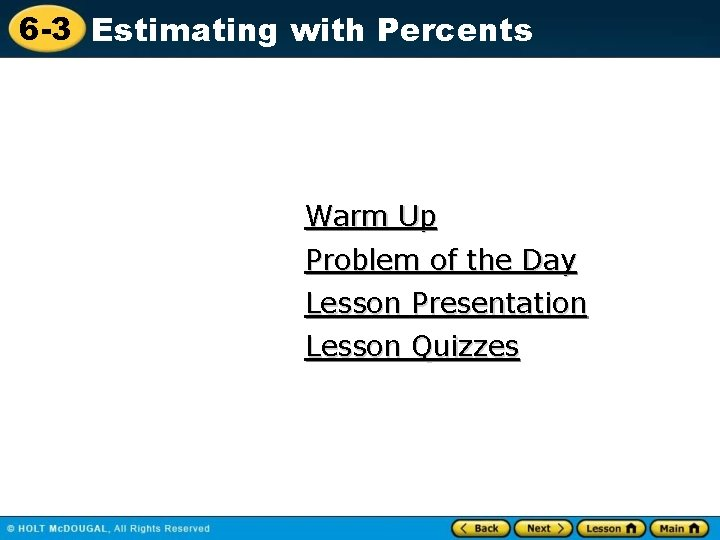6 -3 Estimating with Percents Warm Up Problem of the Day Lesson Presentation Lesson Quizzes6 -3 Estimating with Percents Warm Up Write each percent as a fraction and as a decimal. 1. 75% 3, 0. 75 4 2. 10% 1 , 0. 1 10 3. 32% 8 , 0. 32 25 4. 17% 17 , 0. 17 1006 -3 Estimating with Percents Problem of the Day You are biking to the library. When you are 75% of the way there, you realize you forgot a book. So you turn around 1 and head back. When you are of the 3 way back you realize you don’t need the book, so you turn around again and bike 3. 2 miles back to the library. How far do you live from the library? 6. 4 miles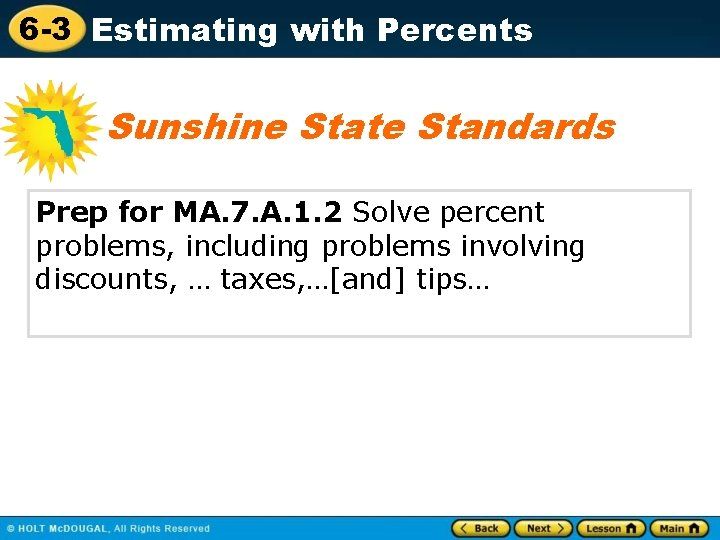6 -3 Estimating with Percents Sunshine State Standards Prep for MA. 7. A. 1. 2 Solve percent problems, including problems involving discounts, … taxes, …[and] tips…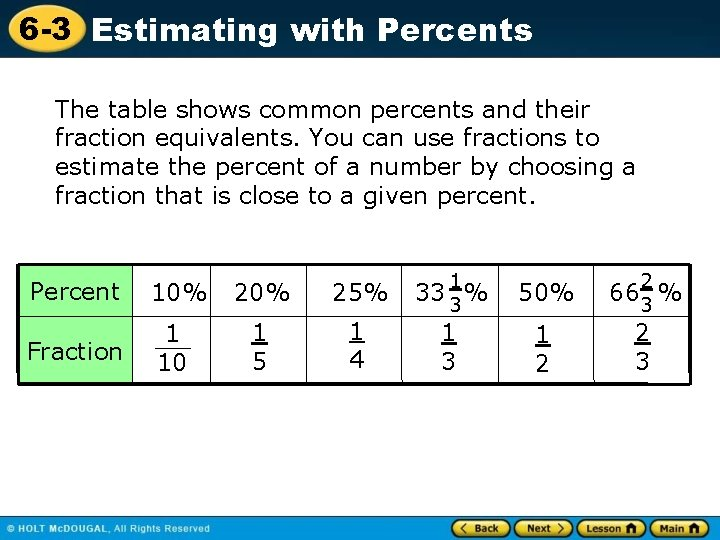6 -3 Estimating with Percents The table shows common percents and their fraction equivalents. You can use fractions to estimate the percent of a number by choosing a fraction that is close to a given percent. Percent 10% 25% Fraction 1 10 1 5 1 4 1 33 3 % 50% 2 663 % 1 3 1 2 2 3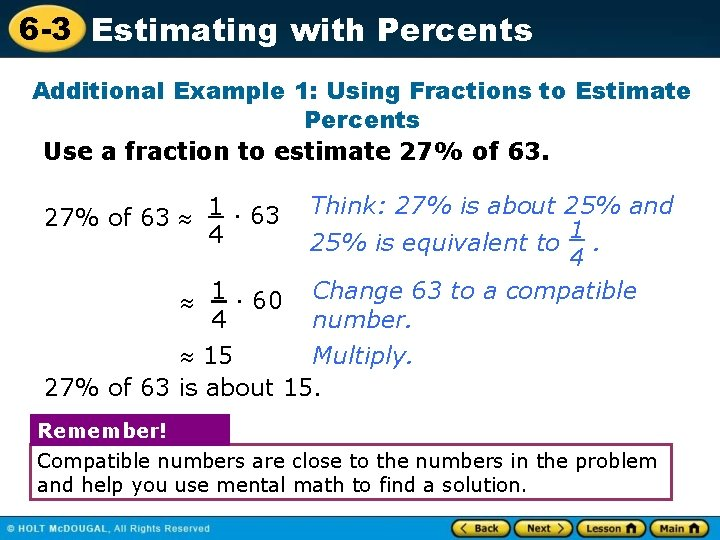6 -3 Estimating with Percents Additional Example 1: Using Fractions to Estimate Percents Use a fraction to estimate 27% of 63. Think: 27% is about 25% and 1 25% is equivalent to. 4 1 · 60 Change 63 to a compatible 4 number. Multiply. 15 27% of 63 is about 15. 27% of 63 1 · 63 4 Remember! Compatible numbers are close to the numbers in the problem and help you use mental math to find a solution.6 -3 Estimating with Percents Check It Out: Example 1 A Use a fraction to estimate the percent of each number. 48% of 81 1 · 81 2 1 · 80 2 40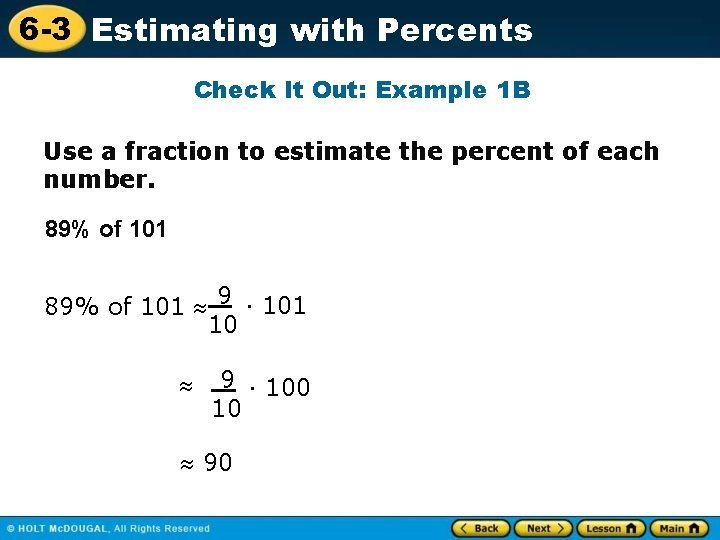6 -3 Estimating with Percents Check It Out: Example 1 B Use a fraction to estimate the percent of each number. 89% of 101 9 · 101 10 9 · 100 10 906 -3 Estimating with Percents Check It Out: Example 1 C Use a fraction to estimate the percent of each number. 33% of 57 1 · 60 3 206 -3 Estimating with Percents Check It Out: Example 1 D Use a fraction to estimate the percent of each number. 12% of 5, 005 1 · 5, 000 10 5006 -3 Estimating with Percents Additional Example 2: Consumer Math Application Tara’s T’s is offering 2 T-shirts for \$18, while Good-T’s is having a sale in which you buy one T-shirt for \$9. 99 and get 30% off a second T-shirt. Which store offers the better deal? First find the discount price for 2 t-shirts at Good T’s. 30% of \$9. 99 = 3 · \$9. 99 Think: 30% is equivalent to 3. 10 10 1 · \$9. 99 Change 3/10 to a compatible number. 3 Multiply. \$3. 33 The second shirt costs approximately \$3. 33. Since \$10 + \$3 = \$13, the 2 T-shirts at Good-T’s is the better deal.6 -3 Estimating with Percents Check It Out: Example 2 Billy’s Office Supply Store is offering 25% off a leather notebook, originally priced at \$9. 75. K’s Office Supply Store offers the same notebook, not on sale, for \$7. 00. Which store offers the better deal? 25% of \$9. 75 25% of 10 1 · 10 4 2. 50 9. 75 - 2. 50 = 7. 50 Price at Billy’s office supply; \$7. 50 Price at K’s office supply; \$7 7. 50 > \$7 so K’s Office Supply Store offers a better deal.6 -3 Estimating with Percents Another way to estimate percents is to find 1% or 10% of a number. You can do this by moving the decimal point in the number. 1% of 45 =. 45. 10% of 45 = 45. . To find 1% of a number, move the decimal point two places to the left. To find 10% of a number, move the decimal point one place to the left.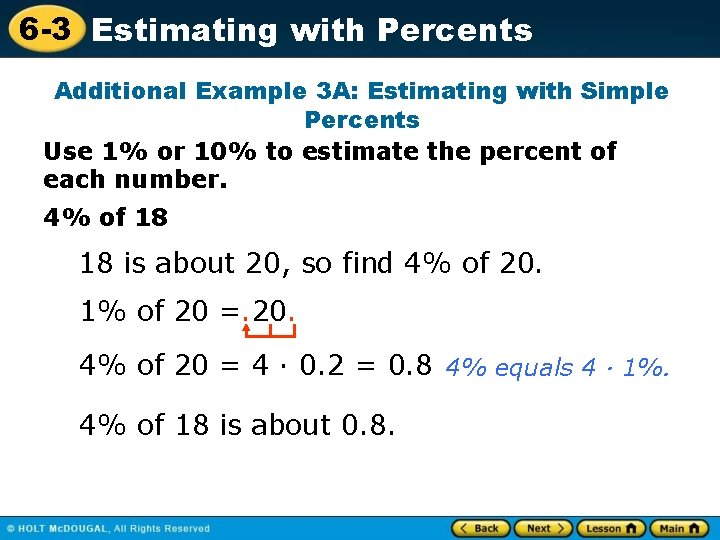6 -3 Estimating with Percents Additional Example 3 A: Estimating with Simple Percents Use 1% or 10% to estimate the percent of each number. 4% of 18 18 is about 20, so find 4% of 20. 1% of 20 =. 20. 4% of 20 = 4 · 0. 2 = 0. 8 4% equals 4 · 1%. 4% of 18 is about 0. 8.6 -3 Estimating with Percents Additional Example 3 B: Estimating with Simple Percents Use 1% or 10% to estimate the percent of each number. 29% of 80 29% is about 30, so find 30% of 80. 10% of 80 = 80. . 30% of 80 = 3 · 8. 0 = 24. 0 30% equals 3 · 10%. 29% of 80 is about 24.6 -3 Estimating with Percents Check It Out: Example 3 A Use 1% or 10% to estimate the percent of each number. 21% of 60 21% is close to 20% 10% of 60 = 6 20% of 60 = 2 · 6 = 12 about 126 -3 Estimating with Percents Check It Out: Example 3 B Use 1% or 10% to estimate the percent of each number. 3% of 29 29 is close to 30 1% of 30 = 0. 3 3% of 30 = 3 · 0. 3 = 0. 9 about 0. 96 -3 Estimating with Percents Additional Example 4: Consumer Math Application Tim spent \$58 on dinner for his family. About how much money should he leave for a 15% tip? Since \$58 is about \$60, find 15% of \$60. 15% = 10% + 5% 10% of \$60 = \$6 Think: 15% is 10% + 5%. 1 2 5% is of 10% so divide 5% of \$60 = \$6 ÷ 2 = \$3 \$6 by 2. \$6 + \$3 = \$9 Add the 10% and 5% estimates. Tim should leave about \$9 for a 15% tip.6 -3 Estimating with Percents Check It Out: Example 4 Amanda spent \$28. 80 on a hair cut. About how much money should she leave for a 15% tip? Since \$28. 80 ≈ \$30, find 15% of 30. 15% = 10% + 5% 10% of 30 = 3. 00 5% of 30 = 3. 00 ÷ 2 = 1. 50 3. 00 + 1. 50 = \$4. 50 tip about \$4. 506 -3 Estimating with Percents Lesson Quizzes Standard Lesson Quiz for Student Response Systems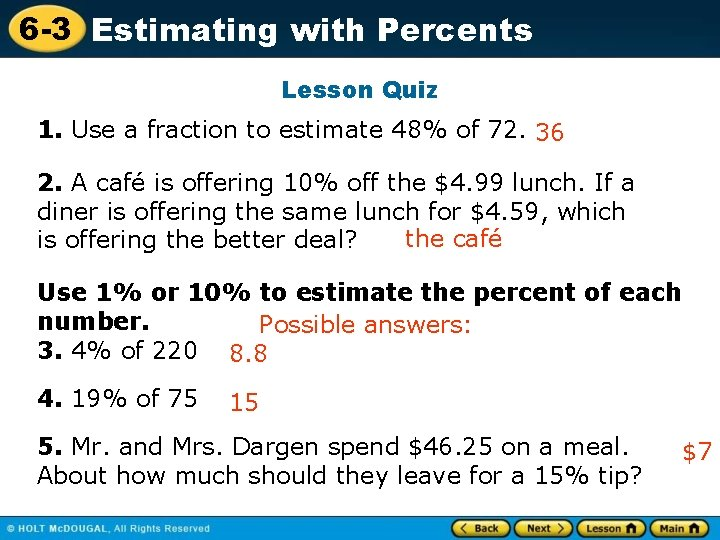6 -3 Estimating with Percents Lesson Quiz 1. Use a fraction to estimate 48% of 72. 36 2. A café is offering 10% off the \$4. 99 lunch. If a diner is offering the same lunch for \$4. 59, which the café is offering the better deal? Use 1% or 10% to estimate the percent of each number. Possible answers: 3. 4% of 220 8. 8 4. 19% of 75 15 5. Mr. and Mrs. Dargen spend \$46. 25 on a meal. About how much should they leave for a 15% tip? \$7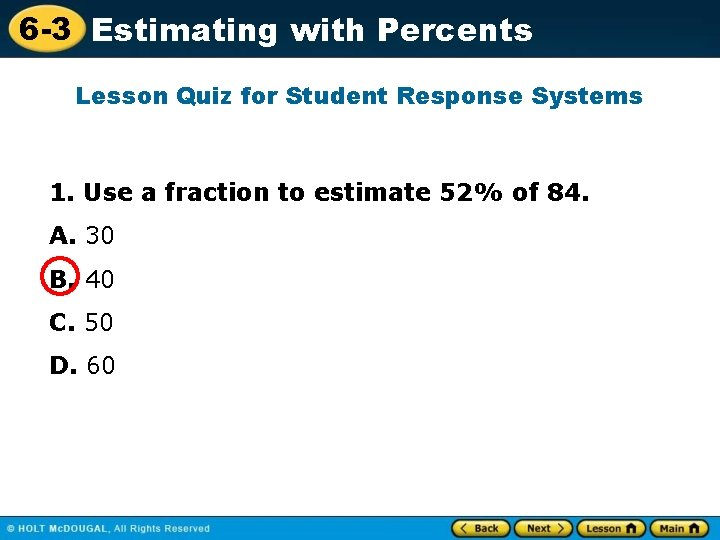6 -3 Estimating with Percents Lesson Quiz for Student Response Systems 1. Use a fraction to estimate 52% of 84. A. 30 B. 40 C. 50 D. 60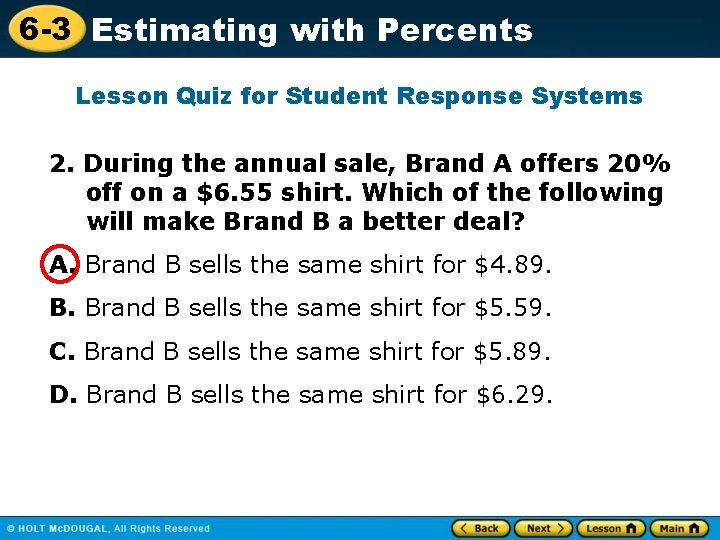6 -3 Estimating with Percents Lesson Quiz for Student Response Systems 2. During the annual sale, Brand A offers 20% off on a \$6. 55 shirt. Which of the following will make Brand B a better deal? A. Brand B sells the same shirt for \$4. 89. B. Brand B sells the same shirt for \$5. 59. C. Brand B sells the same shirt for \$5. 89. D. Brand B sells the same shirt for \$6. 29.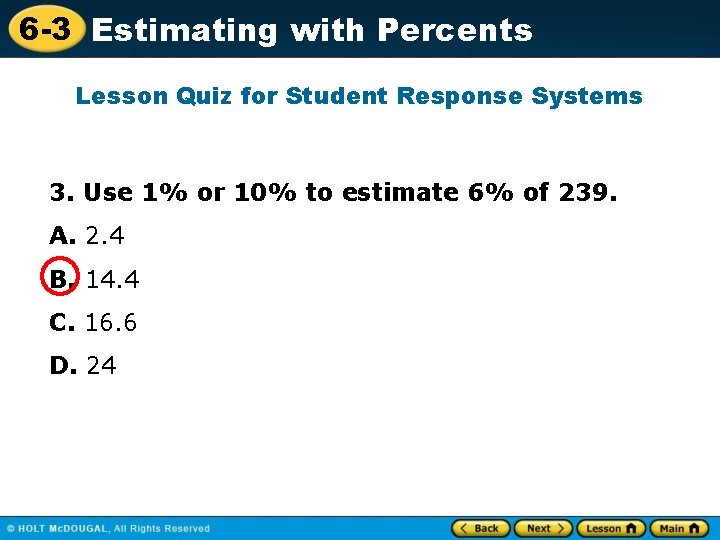6 -3 Estimating with Percents Lesson Quiz for Student Response Systems 3. Use 1% or 10% to estimate 6% of 239. A. 2. 4 B. 14. 4 C. 16. 6 D. 246 -3 Estimating with Percents Lesson Quiz for Student Response Systems 4. Use 1% or 10% to estimate 18% of 88. A. 8. 8 B. 14 C. 18 D. 22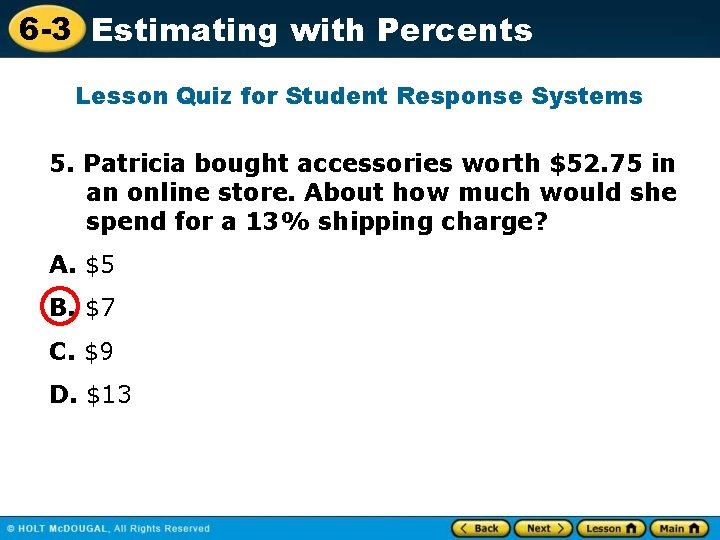6 -3 Estimating with Percents Lesson Quiz for Student Response Systems 5. Patricia bought accessories worth \$52. 75 in an online store. About how much would she spend for a 13% shipping charge? A. \$5 B. \$7 C. \$9 D. \$13